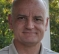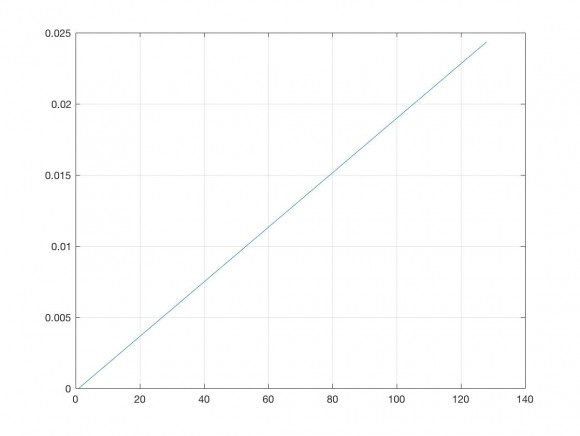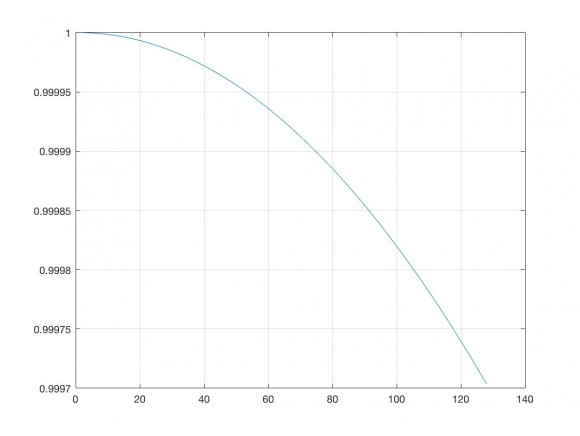## DSBSC DemodulatorStarted by 6 years ago21 replieslatest reply 6 years ago140 views

Hi,

I implemented a dsbsc demodulator using 2nd order costas loop on SoC c5505 eZdsp.

My demodulation functions takes about 45 msec of cpu time, while data arrive from codec every 2 msec.

I need to demodulate the signal without glitches due to this time delay.

I have implemented triple buffering scheme using dma to collect data and transmit to the codec, but it is not enough.

I'm searching desperately any suggestion or tip.

Paolo

[ - ]If i create a receive buffer, long enough, and elaborate then transmit,

i'll transmit with 45 msec delayed, and this isn't a problem.

Agree?

[ - ]Sounds like a software optimization problem to me. Did you try to increase the optimization level of the compiler - does that minimize your 45msec some?

[ - ]Yes, I done, but the time gain is not enough.

I decided to maintain an input queue and an output queue.

When there is a new frame in the input queue, i process it.

Then i put the result in the tail of the output queue.

This should allow me to transmit with 45 msec delay the processed signals in the right order, that in communications is an acceptable delay.

[ - ]Have you run a profile of your code to see where the major real time is consumed?

Can you "compromise" the algorithms to reduce their real time and possibly their performance?

Things like sqrt() are the first places to look.

David

[ - ]Hi David,

I measured a lot of time spent in cos(), sin() and atan2.

Furthermore, I'm implementing my algo with float data, instead of using integers.

Being a newby in fixed point DSPs, it is difficult for me to pass to int operations, but I'll try. My fear is to compromise the precision of the algorithm, reducing the quality of the transmission.

Thanks a lot David

PMartin

[ - ]Wow! Your code is ripe for some clever stuff.

Don't be scared of fixed point math.

Sin and cos in 4 multiplies and 2 adds etc.

Keep angle as binary between 0 and 0x7FFF representing 2xPI.

David

[ - ]Thanks David,

I'm passing from fp to 32 bit integer operations.

How can i convert a float into Q31?

Reasoning it should be:

Q31 = float * 2^31, so Q31 = float << 31

Converting into q15 using fltoq15 (dsplib) i have a down-saturation.

Thanks a lot

PMartin

[ - ]My advice, is not to try to convert from float to fixed.  Rather, start in the fixed point domain.

For the sin and cos, start with the identity sin(a+b) = sin(a)*cos(b)+cos(a)*sin(b). (similarly for cos(a+b))  Now the angle is set up to be in the range 0-0x7fff representing 0-2*pi.

Calculate a table for the sin(a) and cos(a) (remembering that cos(a) = sin(a+pi/2)), where "a" is the upper 8 bits of the angle.  Use shifts (>>7) to retrieve the index into the table.  The table will be 1.25 cycles of a sine wave in 256+256/4 entries.  The cos(a) value is retrieved by simply adding 256/4 = 32 to the "a" index and accessing the table again.

Create another 2 tables that are 128 entries for the sin and cos of the LSBs of the angle.  Range for each is 0-2*pi/256.  Mask the angle with 0x7f to index into sin(b) and cos(b).  The cos terms will all be very close to 1.0.  The sin terms will have a fairly steep climb.

Total 3 tables: 1 course angles including sine and cosine, and two separate for small angles for cosine and sine independently.

Use the trig identities above to calculate the total results for sin(a+b) and cos(a+b).  Total 1 shift, 1 add, 1 mask, 4 lookups, 4 multiplies and 2 adds for sine and cosine.

Now for the angle, everything is nice, because you can increment the angle and wrap using a mask to force the 0-2*pi range.  Has only positive values, so index extraction is shift or mask as mentioned.

The entries in the tables are in Q1.15 format if you are using 16 bit fixed point.  I have used these techniques many times for modems, and the resulting values are totally accurate enough for just about anything you could want.  Error is very small, and angular accuracy is 15 bits or 2*pi/32k.

As the professor would say, "the atan2 is left as an exercise for the student".  I recommend starting with fixed point here too.

One question to always ask is, "how accurate does it really need to be".

Don't go for the textbook answer, and please don't try polynomials in fixed point.  The precision requirements grow way too fast with the order of the polynimial.

Good luck,

David

[ - ]David, you are very very helpful!

I'll try to implement then i'll make you to know

Thanks you so much!

PMartin

[ - ]David, i have some questions:

"Calculate a table for the sin(a) and cos(a) (remembering that cos(a) = sin(a+pi/2)), where "a" is the upper 8 bits of the angle.  Use shifts (>>7) to retrieve the index into the table.  The table will be 1.25 cycles of a sine wave in 256+256/4 entries.  The cos(a) value is retrieved by simply adding 256/4 = 32 to the "a" index and accessing the table again."

Do you mean i should create the table like this:?

sin(0x00), sin(0x01),...,sin(0xFF),sin(0x00),sin(0x01),...,sin(0x1F)?

but 256/4 = 64, not 32 and pi/2 = 0x1F = 32.

"Create another 2 tables that are 128 entries for the sin and cos of the LSBs of the angle.  Range for each is 0-2*pi/256.  Mask the angle with 0x7f to index into sin(b) and cos(b).  The cos terms will all be very close to 1.0.  The sin terms will have a fairly steep climb."

I didn't understand this:

- 1 table for the LSBs of sine

- 1 table for the LSBs of cosine.

why each is 128 entries and not 256 (sin(0x00)..sin(0xFF))?

Thanks

PMartin

[ - ]First, of course you are right that 256/4 is 64.  My mistake.

Next.  The table entries are not calculated from the index, but rather from a fraction of a cycle.

table[0x00] = sin(0), table[0x01] = sin(2*pi(1/256)), table[0x02] = sin(2*pi*(2/256)), table[0x03] = sin(2*pi*(3/256)), ...

The index is 0x00, 0x01, 0x02, 0x03, ... into the table with the above content.  In decimal, we have 0-255 for the course index, and 0-127 for the fine index.

The finer tables are 128 entries

sine_fine_table[0x00] = sin(0),

sine_fine_table[0x01] = sin(2*pi*(1/32768)),

sine_fine_table[0x02] = sin(2*pi*(2/32768)), ...

Equivalent for cosine_fine_table[] with 128 entries.

The angle is in the range 0-0x7fff.  If I shift by 7 bits to the right, in fixed point, then I have the range 0-0xff as index into the course table.  If I mask the full angle with 0x7f, I retrieve the lowest 7 bits as an index into the fine sine and fine cosine tables.[ - ]Very clear.

Obviously, i must use fltoq15 of DSPLIB to convert of the course and fine tables to Q15 format.

Now, how can i apply sin(a+b) = sin(a)cos(b) + sin(b)cos(a), or

cos(a+b) = cos(a)cos(b) - sin(a)sin(b) for the cosine,

What are sin(a) and sin(b)?

Thanks a lot

PMartin

[ - ]Hi again.

Again angle takes on values between 0x0000 and 0x7fff.

0x7fff represents 2*pi - epsilon.

Remember that angle "value" = "value" & 0x7f80 + "value" & 0x7f.

sin((angle & 0x7f80) + (angle & 0x7f)) = course_table[angle>>7] * cosine_fine_table[angle & 0x7f] + course_table[(angle>>7) + 64] * sine_fine_table[angle & 0x7f];

Equivalent for the cosine(angle) using its appropriate identity.

[ - ]Thanks!

I'll try to implement

Best Regards,

PMartin

[ - ]Hi David,

i've implemented sin and cos and i gained 6000 cycles!

I need to exercise in Fixed Point arithmetic to proceed...

I have overflows and underflows in my algorithm, so i need to learn a bit.

Have you some tutorial to suggest?

Thanks a lot!

PMartin

[ - ]Hi,

What percentage of your total real time is 6000 cycles?

I don't have a tutorial for you, I'm afraid.  Most of the hard learn comes from experience.

Overflows are mostly dealt with by saturation.  I'm not sure where the underflows would come from with DSBSC, except maybe for some filtering.  I presume from your comment, that the precision is satisfactory for your needs.

Anyway, it sounds like you are on you way to becoming a fixed point practitioner.

David

[ - ]Yes,

6000 cycles is an important gain. I gain them in a 384 loop, so i gain, at 120 Mhz about 19 msec only by sine and cosine.

Thanks a lot

PMartin

[ - ]Just a little less than half of the 45 msec?

That is pretty good.  I suspect that atan2 will bring you a lot of savings too.

[ - ]Yes, a lot is gained from sums and multiplications too...

apart the fact of overflows and underflows said before...

[ - ]Hi David,

there is something wrong in my implementation.

I tested the algo calculating sine and cosine of 5/6*pi.

If 2*pi = 0X7FFF (32767), 5/6*pi = 13653.

Calculations of sine and cosine are wrong:

sine: 8552 ==> 0.260986328125 instead of 0.5

cosine: 7168 ==> 0.21875 instead of -0.8660254

Here is my implementation:

#define w_coarse 0.0245437

#define w_fine   0.0001917

void prepare_course_tab(void)

{

Uint16 i;

for(i = 0 ; i < 320; i++)

course_tab[i] = sin(i*w_coarse);

fltoq15(course_tab,course_tab_Int,320);

}

void prepare_sine_fine_tab(void)

{

Uint16 i;

for(i = 0 ; i < 128; i++)

sin_fine_tab[i] = sin(i*w_fine);

fltoq15(sin_fine_tab,sin_fine_tab_Int,128);

}

void prepare_cosine_fine_tab(void)

{

Uint16 i;

for(i = 0 ; i < 128; i++)

cos_fine_tab[i] = cos(i*w_fine);

fltoq15(cos_fine_tab,cos_fine_tab_Int,128);

}

Int16 iSin(Int16 angle){

return  (course_tab_Int[angle>>7] * cos_fine_tab_Int[angle & 0x7f] + course_tab_Int[(angle>>7) + 64] * sin_fine_tab_Int[angle & 0x7f]);

}

Int16 iCos(Int16 angle){

return  (course_tab_Int[(angle>>7) + 64] * cos_fine_tab_Int[angle & 0x7f]  - course_tab_Int[(angle>>7)] * sin_fine_tab_Int[angle & 0x7f]);

}

I have checked the fine tables and i found your description:

sines are ramped up, cosines close to 1.

Thanks a lot

PMartin

[ - ]Hi David, solved:

sum and products in iSin and iCos overflowed:

Here's the solution:

Int16 iSin(Int16 angle){
Int16 cc;  // cosine course
Int16 cf;  // cosine fine
Int16 sc;  // sine course
Int16 sf;  // sine fine
Int16 sinacosb;
Int16 sinbcosa;
Int16 sin_angle;
sc = course_tab_Int[angle>>7];
cf = cos_fine_tab_Int[angle & 0x7f];
cc = course_tab_Int[(angle>>7) + 64];
sf = sin_fine_tab_Int[angle & 0x7f];
sinacosb = (((Int32)sc)*((Int32)cf)) >> 15;
sinbcosa = (((Int32)cc)*((Int32)sf)) >> 15;
sin_angle = sinacosb + sinbcosa;
return  sin_angle;
}



Since i need both sine and cosine of the same angle, i gain 20  cycles

void iSinAndCos(Int16 angle, Int16* sin_angle, Int16* cos_angle){
Int16 cc;  // cosine course
Int16 cf;  // cosine fine
Int16 sc;  // sine course
Int16 sf;  // sine fine
Int16 cosacosb;
Int16 sinasinb;
Int16 sinacosb;
Int16 sinbcosa;
sc = course_tab_Int[angle>>7];
cf = cos_fine_tab_Int[angle & 0x7f];
cc = course_tab_Int[(angle>>7) + 64];
sf = sin_fine_tab_Int[angle & 0x7f];
sinacosb = (((Int32)sc)*((Int32)cf)) >> 15;
sinbcosa = (((Int32)cc)*((Int32)sf)) >> 15;
cosacosb = (((Int32)cc)*((Int32)cf)) >> 15;
sinasinb = (((Int32)sc)*((Int32)sf)) >> 15;
*sin_angle = sinacosb + sinbcosa;
*cos_angle = cosacosb - sinasinb;
}



Hi David

Best Regards

PMartin